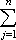#Interactive Real Analysis

Next | Previous | Glossary | Map

## 7.1. Riemann Integral

### Definition 7.1.3: Riemann Sums

If P = { x0, x1, x2, ..., xn} is a partition of the closed interval [a, b] and f is a function defined on that interval, then the n-th Riemann Sum of f with respect to the partition P is defined as:
R(f, P) =f(tj) (xj - xj-1)
where tj is an arbitrary number in the interval [xj-1, xj].

Note: If ti is always the left endpoint of each subinterval, the corresponding Riemann sum is called left Riemann sum; if it is always the right endpoint, the sum is called right Riemann sum.

Next | Previous | Glossary | Map